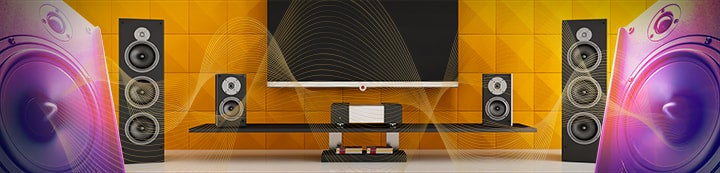arithmetic
1-9 of 9 Results
1. ### [REQ] preserve ref time on trace arithmetic (or add a copy function)

REW Forum
atm you can copy a trace using (A+B)/2 but the copied measurement is left with ref time of t=0 no matter where the original traces are. It would be nice if this were set automatically and/or an explicit copy option were added that avoided the need to use trace arithmetic at all. On a related...
2. ### trace arithmetic and average the response

REW Forum
Hi all, I have a question: what is the difference between the trace arithmetic function A+B and the button at the bottom left of the graphic named average the response ? The graphs are very similar but with different values, is it a geometrical average ? Also, what is the difference between A+B...
3. ### Does trace arithmetic work correctly with many measurements?

REW Forum
This might be a stupid question but I'll ask anyway. REW lets you sum 2 traces but say I want to sum multiple sources (e.g. >2 subs), does this work correctly in REW? i.e. does A + B + C = (A + B) + C when it comes to summing measured responses?
4. ### Measurement arithmetic functions ?

REW Forum
Hi all how do i do to merge/add/divide measurements in REW ? (i want to average 4 impulse responses ) thanks
5. ### Arithmetic inversion suggestion

REW Forum
Would it be possible to include arithmetic inversion (B = 1 / A) in the future REW versions?
6. ### Trace Arithmetic

REW Forum
Hi I am trying to make a calibtration file for REW. With the facility Trace Arithmetic how would i do this.? i have tried to do this with the different calculations then import that file, but it doesnt work. I have attached the txt files for both mics, both named. Your help would be...
7. ### trace arithmetic

REW Forum
I had measured the woofer and mid driver response separately and combined them using trace arithmetic (A+B) and am puzzled by the results. see attached screen shot. at freq = 212Hz, amplitudes from the graph are: A=125.1, B=125.5 A+B=98.7db From my calculation...
8. ### Newbie - Where is arithmetic tab?

REW Forum
I am running REW V5 on a Vista laptop. Everything seems to work fine - testing line level crossover signal from my receiver. I can't find the arithmetic tab though. I wanted to sum 2 curves, and after looking over the help manual, it looks like it should be an option under "Overlays". When I...
9. ### Running EQ module for Arithmetic generated trace

REW Forum
Hi All, Is it possible to run EQ filters synthesis for calculated arithmetic traces? I see no restrictions about this in Help file, I tried to do this but no luck. Steps to reproduce: - make two measures (dummy ones), call it "1" and "2". - make trace "3" by generating trace in "Trace...
1-9 of 9 Results